Next: Algorithms for algebraic pattern Up: Heuristic Algorithms Previous: Signatures of sets

# Computation with algebraic numbers

The problem that we want to address here is how to compute effectively with algebraic numbers and algebraic functions. Part of the algorithms presented here were studied and developed by Trevor J. Smedley for his thesis dissertation. His thesis and related papers are the main references for this chapter.

What do we mean by computation with algebraic numbers?

• Normalizing expressions, i.e. representing every algebraic number of arithmetic expression involving algebraic numbers, into a canonical, simplified form. For algebraic numbers of order k, all powers k or larger will be reduced.
• Rationalize expressions. Remove the occurrence of the algebraic numbers in the denominator of expressions.
• Gcd computations.
• Polynomial factorization.
• Other polynomial operations, e.g. resultants.

There are deterministic conventional algorithms for all of the above problems, but these are, in some cases, very difficult to implement and always extremely expensive to run.

The heuristic algorithms presented in this chapter are two variations of the same flavour. In both cases we reduce our domain from polynomials over the integers with the addition of algebraic numbers to a domain of polynomials over the integers mod n and without algebraic numbers. In the first method, the one described in Smedley et al., we select the representation of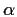so that it is easy to lift the results. For the second method, we rely on the LLL algorithm for lifting the results. Complete algorithms for the first method are currently implemented and used in Maple.

Letbe an algebraic number defined by the polynomial p(x) with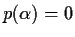. Without loss of generality we will assume that p(x) is an primitive, irreducible polynomial over the integers of degree 2 or higher. The degree of the algebraic number, is the degree of the polynomial,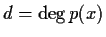. If the leading coefficient of p(x) is 1 or -1, thenwill be called an algebraic integer.

From the above definitions and the proposed mapping, it is obvious that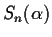should be selected so that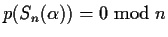, or thatis a root of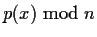(or that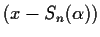is a factor of).

Example. Suppose we want to simplify, whereis an algebraic number of degree 3, i.e. d = 3. We know that the answer will be of the form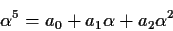If we chooseto be a root of, the resulting signature foris typically a number as large as n and it is not posible to easily retrieve a0, a1 and a2. Using the same ideas that we used in GCDHEU, we should try to obtain anwhich is very small with respect to n, so that then the coefficients ai can be lifted with the mods algorithm described before.

It is not very difficult to find an n such that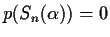for small. But first lets be a bit more precise. Lets assume that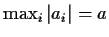. Sojust to be able to lift the coefficients. In particular,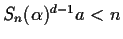to be able to lift the last coefficient. In summary this means that

(2a)d < 2n

The procedure to selectand n witht the above conditions is as follows:

You can experiment with other values for a by changing its assignment, or with other expressions inby changing the assignment to
tt S.

There is an alternative approach to solve this problem which does not require the searching of a suitable prime (which in turns requires a partial factorization). This alternative also resolves the problem of common denominators which are very difficult to estimate correctly. It is based on the LLL algorithm and proceeds as follows.

For some arbitrary expression, e involving an algebraic number, we compute its signature using some prime number n. This prime number n is chosen so that the characteristic polynomial p(x) has at least one linear factor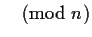. (Obtaining the linear factors of a polynomialis much easier than factoring.) We assume that our expression e can be expressed in terms of the algebraic numberas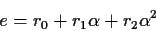for rational numbers ri (usually with a common denominator). For our use of the LLL algorithm, we assume that these rirationals are composed of small'' numerators and denominators, at least small compared with n. The matrix is set up as usual. The first column should satisfy the equality of the signature of e and the signature of the expression in the ri. We multiply this column by a large integer to insure that most of the answer vectors will have a 0 (exact satisfaction) in this position. The second column will have the multiplier of the signature of e, that will play the role of the common denominator of all the ri. The remaining colums give the numerators of the ri.Next: Algorithms for algebraic pattern Up: Heuristic Algorithms Previous: Signatures of sets
Gaston Gonnet
1999-07-04